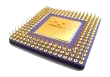CDSP-k5 Performance Benchmark suite for common DSP algorithmsThe following table presents a performance estimation for some common DSP algorithms.
The estimation is based on a fully-featured architecture that includes the complete MAC unit and supports the post-increment and bit-reverse indexing facilities.

 Operation Description Conditions Parameters Cycle-Count Complex FIR Finite Impulse Response Filter with Constant or Variable Complex Coefficients Arbitrary number of Coefficients and Samples N=number of coefficients  M=number of samples M*(N+p)+q Real FIR Finite Impulse Response Filter with Constant or Variable Real Coefficients Even number of Coefficients N=number of coefficients  M=number of samples M/2*(N+p)+q Complex IIR Direct Form II IIR Filter, Constant or Variable Complex Coefficients Cascaded biquad sections, Samples multiple of 8 N=number of samples  M=number of cells N/A LMS Complex FIR Coefficients Update Update the Complex FIR coefficients using the Least Mean SquareAlgorithm Even number of coefficients N=Number of coefficients 2*N+p Complex Autocorrelation Complex Autocorrelation Arbitrary input and output vector size N=number of input samples  M=number of output samples M*(N+p)+q Real Autocorrelation Real Autocorrelation Even number of samples N=number of input samples  M=number of output samples M/2*(N+p)+q Complex Energy Square each complex element in a vector and sum all the squared values Arbitrary vector size N=vectors size N+p Real Energy Square each real element in a vector and sum all the squared values Even number of samples N=vectors size N/2+p Complex FFT Radix 2 Fast Fourier Transform Number of samples is a power of 2 N=number of samples Log2(N)*(2*N+p)+q Complex Dot Product Point-by-point multiplication of two equal-size complex vectors Arbitrary vector size N=vector size 2*N+p Real Dot Product Point-by-point multiplication of two equal-size real vectors Even number of samples N=vector size N+p Complex Weighted Vector Sum Add two complex vectors elements having one of them multiplied by a complex constant Even number of elements in the vectors N=vectors size 3*N+p Real Weighted Vector Sum Add two real vectors elements having one of them multiplied by a real constant Even number of elements in the vectors N=vectors size 3*N/2+p Real Max/Min Find the minimum/maximum value in a vector and retainits position Arbitrary vector size N=vectors size N+p Real or Complex Search/Skip Search until/while a value is found in a vector and retain the (last) position where it was found Arbitrary vector size N=vectors size N+p Complex Move Move a complex vector from one position to another in memory Arbitrary vector size N=vectors size 2*N+p Real Move Move a real vector from one position to another in memory Even number of samples N=vectors size N+p

Note 1: "p" and "q" count for the overhead loop-initialization instructions. Performance significantly degrades when "N" is relatively small(<32) as compared with "p".